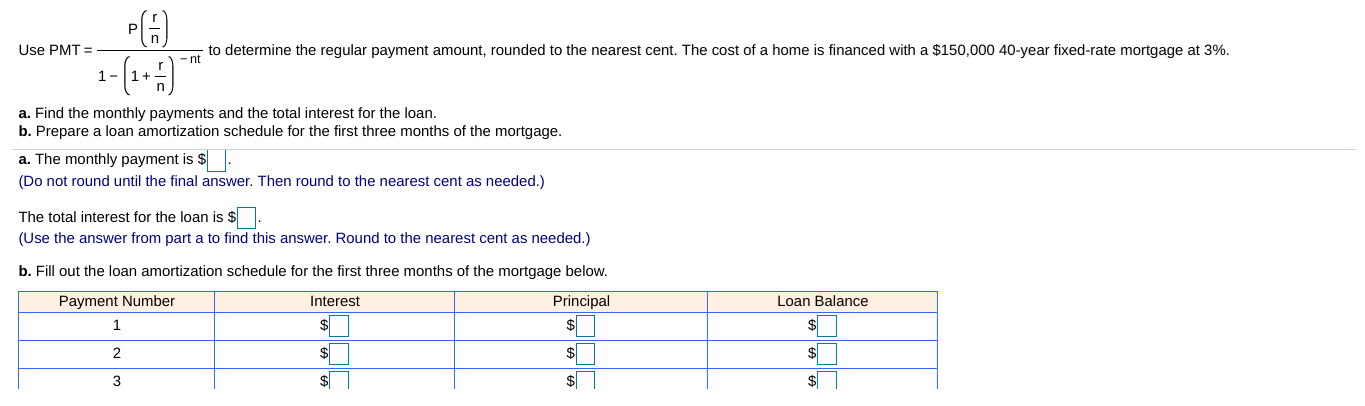# Use PMTto determine the regular payment amount, rounded to the nearest cent. The cost of a home is financed with a \$150,000 40-year fixed-rate mortgage at 3%a. Find the monthly payments and the total interest for the loan.b. Prepare a loan amortization schedule for the first three months of the mortgage.a. The monthly payment is \$Do not round until the final answer. Then round to the nearest cent as needed.)The total interest for the loan is s(Use the answer fom part a to find this answer. Round to the nearest cent as needed.)b. Fill out the loan amortization schedule for the first three months of the mortgage below.PrincipalLoan BalancePayment NumberInterest

Question
250 views

on B (Use the answer from part a to find these answers. Round to the nearest cent as​ needed.)help_outlineImage TranscriptioncloseUse PMT to determine the regular payment amount, rounded to the nearest cent. The cost of a home is financed with a \$150,000 40-year fixed-rate mortgage at 3% a. Find the monthly payments and the total interest for the loan. b. Prepare a loan amortization schedule for the first three months of the mortgage. a. The monthly payment is \$ Do not round until the final answer. Then round to the nearest cent as needed.) The total interest for the loan is s (Use the answer fom part a to find this answer. Round to the nearest cent as needed.) b. Fill out the loan amortization schedule for the first three months of the mortgage below. Principal Loan Balance Payment Number Interest fullscreen
check_circle

Step 1

P = \$ 150,000

r = 3% = 0.03

n = 12 (months in a year)

t = 40 years

Step 2

(a)

The monthly payment = PMT = \$ 536.98

Step 3

Total interest payment = Total payment - Principal = PMT x n x t - P = 53...

### Want to see the full answer?

See Solution

#### Want to see this answer and more?

Solutions are written by subject experts who are available 24/7. Questions are typically answered within 1 hour.*

See Solution
*Response times may vary by subject and question.
Tagged in